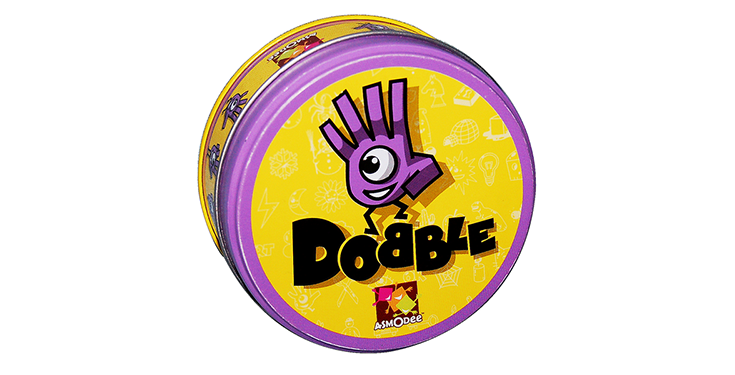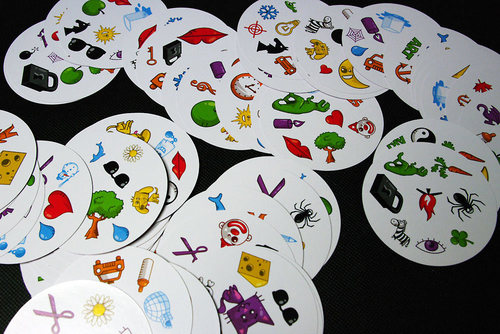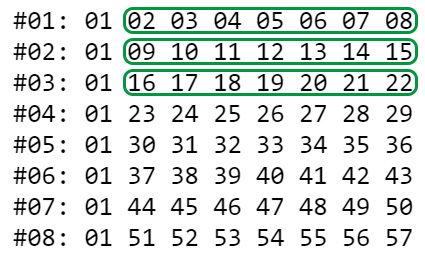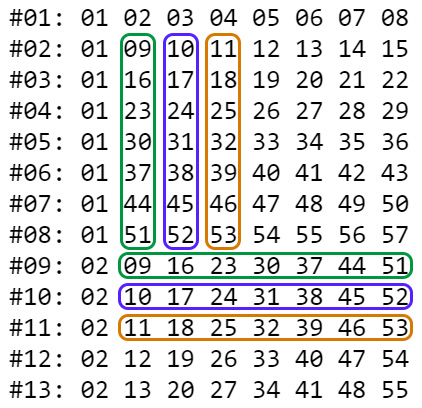# Algorithm and JavaScript function for Dobble (Spot it!) gameA JavaScript function to generate combinatorial geometric series for games such as Dobble (Spot it!) and to better understand the math logic behind themHave you ever heard of Dobble? If you have children, I bet you did! Anyway, it’s a pattern recognition board game for children (but also great for all ages) where the players must idenfity common shapes across multiple cards as quickly as possible.

The game has 55 cards, each of which presents 8 different shapes arranged in random order. The game is made so that each pair of cards has one (and only one) shape in common, which players must find as soon as possible. The game was released in 2009 under the name Spot it!, while Dobble is an alternative market name mostly used in European countries (including Italy, where it was published by Asmodee around 2013). For more information about this game, we strongly advise to take a look at its page on the BoardGameGeek community website.If you came across this article, it most likely means that you’re interested in learning more about how the game’s creators managed to made Dobble cards so that each of them always has one (and only) shape in common with all the others. In this article we’ll try to understand how they did that thanks to a lightweight JavaScript function that can be used to achieve the same result, even with a different number of cards and/or symbols. Such function could also be used by anyone to create their own customized version of Dobble.

## A “geometric” problem

As you can easily imagine, the underlying logic that determines the set of shapes printed on each card is the result of an algorithm of some sort. More specifically, such assignment logic can be calculated with a function able to generate combinatorial geometric series with the following characteristics:

• each series must have a specific number (N) of different element (taken from a common element pool);
• each series must have one (and only one) element in common with each other series;

In the case of Dobble, each series (the card) is composed of 8 elements (the shapes): N is therefore equal to 8. We also know that the deck is made of 55 cards. Therefore, our function must be able to produce (at least) 55 different series with 8 internally-different elements and 1 (and only one) element in common with any other card.

## The source code

The dobble() function in the JavaScript code below can be used to generate the maximum number of series (cards) with a given N number of elements: however, the algorithm will only work if N has a value of any prime number +1. It could be 8 (as in Dobble) or also 3, 4, 6, 12, 14, 18, 20, and so on. If an invalid N value is used, the function will show a warning – and the outputTests() testing function will have some failures.

It goes without saying that each number contained in the various series is meant to represent a unique shape among those present on our cards: the number 1 for the heart, the number 2 for the tree, and so on. For the sake of simplicity, we won’t make the numbers-to-shapes mapping in this post, being it a rather trivial task.

The version in this article might be obsolete: the most up-to-date build is available on GitHub.

To try this function and/or change its values to generate different series, check out this JSFiddle.

As we can see, the function performs two cycles: the first constructs a serial number equal to the number of elements (N) by placing the element number 1 in the first position and then filling them with the other elements in ascending order by following a horizontal progression (from left to right); such logic will make sure that all series produced so far will have the element number 1 (and only that one) in common. This operation, as can be seen from the image, also constructs a matrix that contains all the elements.The second cycle builds all the remaining series by placing the elements number 2, 3, 4, 5 and so on (up to N) in the first position and then filling them with the other elements in ascending order: however, this time the progression develops vertically (from top to bottom) to be sure to “intersect” once (and only once) any series generated during the previous cycles.Also, whenever the number in the first position changes, the vertical progression path is incrementally shifted to the right of the matrix starting from the number in position 3, so that each subsequent series will only “intersect” each vertical column of the matrix once (and only once).

By looking at how the above cycles work, we can easily understand how the maximum number of different series that this function can produce is equal to N + (N-1) * (N-1). In the case of Dobble, where N is equal to 8, it means that we can have up to a maximum of 8 + (8-1) * (8-1) = 57 different cards: that’s 2 more than the number of cards contained in the Italian edition! If the shapes on each card were 10, we could have up to 91; if they were 12, up to 133; and so on. Just remember that N must be a prime number +1, otherwise you’ll end up with a certain amount of invalid series (that will be shown by the testing function ouput).

## Conclusion

If you like this script, feel free to give me a virtual hug by starring the GitHub project and/or putting a like on our Facebook/Twitter pages!